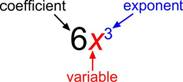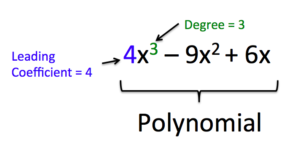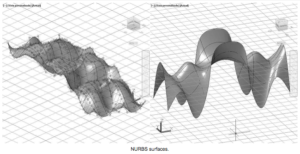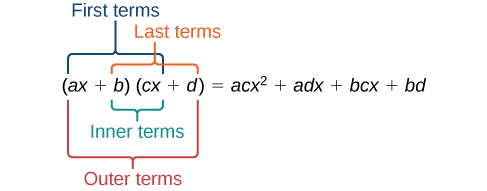## 7.1 – Algebraic Operations on Polynomials

### Learning Objectives

• (7.1.1) – Identify the degree and leading coefficient of a polynomial
• (7.1.2) – Evaluate a polynomial for given values
• (7.1.3) – Adding and subtracting of polynomials
• (7.1.4) – Find the product of polynomials
• Find the product of monomials
• Find the product of monomials and polynomials
• Find the product of two binomials using the FOIL method
• Multiply a Trinomial and a Binomial

# (7.1.1) – Identify the degree and leading coefficient of a polynomial

In the example on the previous page, we saw how combining the formulas for different shapes provides a way to accurately predict the amount of paint needed for a construction project. The result was a polynomial.

A polynomial function is a function consisting of sum or difference of terms in which each term is a real number, a variable, or the product of a real number and variables with an non-negative integer exponents. Non negative integers are 0, 1, 2, 3, 4, …
You may see a resemblance between expressions and polynomials, which we have been studying in this course.  Polynomials are a special sub-group of mathematical expressions and equations.

The following table is intended to help you tell the difference between what is a polynomial and what is not.

 IS a Polynomial Is NOT a Polynomial Because $\displaystyle 2x^2-\frac{1}{2}x -9$ $\displaystyle \frac{2}{x^{2}}+x$ Polynomials only have variables in the numerator $\displaystyle \frac{y}{4}-y^3$ $\displaystyle \frac{2}{y}+4$ Polynomials only have variables in the numerator $x^3y^2+4$ $\displaystyle \frac{x^3}{y^2} +4$ Polynomials only have variables in the numerator $\sqrt{12}\left(a\right)+9$ $\sqrt{a}+7$ Roots are equivalent to rational exponents, and polynomials only have integer exponents

The basic building block of a polynomial is a monomial. A monomial is one term and can be a number, a variable, or the product of a number and variables with an exponent. The number part of the term is called the coefficient.A polynomial containing two terms, such as $2x - 9$, is called a binomial. A polynomial containing three terms, such as $-3{x}^{2}+8x - 7$, is called a trinomial.

We can find the degree of a polynomial by identifying the highest power of the variable that occurs in the polynomial. The term with the highest degree is called the leading term because it is usually written first. The coefficient of the leading term is called the leading coefficient. When a polynomial is written so that the powers are descending, we say that it is in standard form. It is important to note that polynomials only have integer exponents.Note, when there is more than one variable in a term, the degree of the term is the sum of all the exponents of all the variables. For example, the degree of the term $4x^3y^2$ is $2+3=5$.

#### Given a polynomial expression in one variable, identify the degree and leading coefficient.

1. Find the highest power of $x$ to determine the degree.
2. Identify the term containing the highest power of $x$ to find the leading term.
3. Identify the coefficient of the leading term.

### DEGREE OF A POLYNOMIAL

The degree of a term is the sum of the exponents of its variables.

The degree of a constant is 0.

The degree of a polynomial is the highest degree of all its terms.

### Example

For the following polynomials, identify the degree, the leading term, and the leading coefficient.

1. $3+2{x}^{2}-4{x}^{3}$
2. $5{t}^{5}-2{t}^{3}+7t$
3. $6p-{p}^{3}-2$
4. $9xy^3+x^3$

In the following video example, we will identify the terms, leading coefficient, and degree of a polynomial.

The table below illustrates some examples of monomials, binomials, trinomials, and other polynomials. They are all written in standard form.

 Monomials Binomials Trinomials Other Polynomials 15 $3y+13$ $x^{3}-x^{2}+1$ $5x^{4}+3x^{3}-6x^{2}+2x$ $\displaystyle \frac{1}{2}x$ $4p-7$ $3x^{2}+2x-9$ $\frac{1}{3}x^{5}-2x^{4}+\frac{2}{9}x^{3}-x^{2}+4x-\frac{5}{6}$ $-4y^{3}$ $3x^{2}+\frac{5}{8}x$ $3y^{3}+y^{2}-2$ $3t^{3}-3t^{2}-3t-3$ $16n^{4}$ $14y^{3}+3y$ $a^{7}+2a^{5}-3a^{3}$ $q^{7}+2q^{5}-3q^{3}+q$ $5m^2n^2$ $n+5n^2m$ $-x^3y^3+xy^2-8y^2$ $x^3y^5+9x^2y^2-8xy+2x+5$

When the coefficient of a polynomial term is 0, you usually do not write the term at all (because 0 times anything is 0, and adding 0 doesn’t change the value). The last binomial above could be written as a trinomial, $14y^{3}+0y^{2}+3y$.

A term without a variable is called a constant term, and the degree of that term is 0. For example 13 is the constant term in $3y+13$. You would usually say that $14y^{3}+3y$ has no constant term or that the constant term is 0.

# (7.1.2) – Evaluate a polynomial for given values

You can evaluate polynomials just as you have been evaluating expressions all along. To evaluate an expression for a value of the variable, you substitute the value for the variable every time it appears. Then use the order of operations to find the resulting value for the expression.

### Example

Evaluate $3x^{2}-2x+1$ for $x=-1$.

### Example

Evaluate $\displaystyle -\frac{2}{3}p^{4}+2^{3}-p$ for $p = 3$.

IN the following video we show more examples of evaluating polynomials for given values of the variable.

# (7.1.3) – Adding and subtracting of polynomials

We can add and subtract polynomials by combining like terms, which are terms that contain the same variables raised to the same exponents. For example, $5{x}^{2}$ and $-2{x}^{2}$ are like terms, and can be added to get $3{x}^{2}$, but $3x$ and $3{x}^{2}$ are not like terms, and therefore cannot be added.

### Example

Find the sum.

$\left(12{x}^{2}+9x - 21\right)+\left(4{x}^{3}+8{x}^{2}-5x+20\right)$

Here is a summary of some helpful steps for adding and subtracting polynomials.

### Given multiple polynomials, add or subtract them to simplify the expressions.

1. Combine like terms.
2. Simplify and write in standard form.

When you subtract polynomials you will still be looking for like terms to combine, but you will need to pay attention to the sign of the terms you are combining. In the following example we will show how to distribute the negative sign to each term of a polynomial that is being subtracted from another.

### Example

Find the difference.

$\left(7{x}^{4}-{x}^{2}+6x+1\right)-\left(5{x}^{3}-2{x}^{2}+3x+2\right)$

In the following two examples, we add and subtract polynomials with more than one variable:

### ExAMPLE

Find the sum $(2x^2-3xy-2y^2)+(5x^2-3xy)$

### ExAMPLE

Find the difference $(p^2+q^2)-(p^2+10pq-2q^2)$

# (7.1.4) – Find the product of polynomials

Multiplying polynomials is a bit more challenging than adding and subtracting polynomials. We must use the distributive property to multiply each term in the first polynomial by each term in the second polynomial. We then combine like terms. First, we review how to multiply monomial by a monomial and a monomial by a polynomial:

### Find the product of monomials

Multiplying polynomials involves applying the rules of exponents and the distributive property to simplify the product. Polynomial multiplication can be useful in modeling real world situations. Understanding polynomial products is an important step in learning to solve algebraic equations involving polynomials. There are many, varied uses for polynomials including the generation of 3D graphics for entertainment and industry, as in the image below.In the exponents section, we practiced multiplying monomials together, like we did with this expression: $24{x}^{8}2{x}^{5}$. The only thing different between that section and this one is that we called it simplifying, and now we are calling it polynomial multiplication.  Remember that simplifying a mathematical expression means performing as many operations as we can until there are no more to perform, including multiplication.  In this section we will show examples of how to multiply more than just monomials.  We will multiply monomials with binomials and trinomials. We will also learn some techniques for multiplying two binomials together.

### Example

Multiply. $-9x^{3}\cdot 3x^{2}$

That’s it! When multiplying monomials, multiply the coefficients together, and then multiply the variables together. Remember, if two variables have the same base, follow the rules of exponents, like this:

$\displaystyle 5{{a}^{4}}\cdot 7{{a}^{6}}=35{{a}^{10}}$

### Example

Multiply. $(-2)x^{3}y^2\cdot (-5)x^{2}y^4$

The following video provides more examples of multiplying monomials with different exponents.

### Find the product of monomials and polynomials

You may have used the distributive property to help you solve linear equations such as $2\left(x+7\right)=21$. We can distribute the $2$ in $2\left(x+7\right)$ to obtain the equivalent expression $2x+14$. When multiplying polynomials, the distributive property allows us to multiply each term of the first polynomial by each term of the second. We then add the products together and combine like terms to simplify.

The following video will provide you with examples of using the distributive property to find the product of monomials and polynomials.

Below is a summary of the steps we used to find the product of two polynomials using the distributive property.

### How To: Given the multiplication of two polynomials, use the distributive property to simplify the expression.

1. Multiply each term of the first polynomial by each term of the second.
2. Combine like terms.
3. Simplify.

### Find the product of two binomials using the FOIL method

We can also use a shortcut called the FOIL method when multiplying binomials. It is called FOIL because we multiply the first terms, the outer terms, the inner terms, and then the last terms of each binomial.The FOIL method arises out of the distributive property. We are simply multiplying each term of the first binomial by each term of the second binomial, and then combining like terms.

### Example

Use FOIL to find the product. $(2x-18)(3x+3)$

In this video, we show an example of how to use the FOIL method to multiply two binomials.

The following steps summarize the process for using FOIL to multiply two binomials.  It is very important to note that this process only works for the product of two binomials. If you are multiplying a binomial and a trinomial, it is better to use a table to keep track of your terms.

### How To: Given two binomials, use FOIL to simplify the expression.

1. Multiply the first terms of each binomial.
2. Multiply the outer terms of the binomials.
3. Multiply the inner terms of the binomials.
4. Multiply the last terms of each binomial.
6. Combine like terms and simplify.

### Multiply a Trinomial and a Binomial

Another type of polynomial multiplication problem is the product of a binomial and trinomial. Although the FOIL method can not be used since there are more than two terms in a trinomial, you still use the Distributive Property to organize the individual products. Using the distributive property, each term in the binomial must be multiplied by each of the terms in the trinomial.

For our first examples, we will show you two ways to organize all of the terms that result from multiplying polynomials with more than two terms. The most important part of the process is finding a way to organize terms.

### Example

Find the product.  $\left(3x+6\right)\left(5x^{2}+3x+10\right)$.

As you can see, multiplying a binomial by a trinomial leads to a lot of individual terms! Using the same problem as above, we will show another way to organize all the terms produced by multiplying two polynomials with more than two terms.

### Example

Multiply. $\left(3x+6\right)\left(5x^{2}+3x+10\right)$

Notice that although the two problems were solved using different strategies, the product is the same. Both the horizontal and vertical methods apply the Distributive Property to multiply a binomial by a trinomial.

In our next example we will multiply a binomial and a trinomial that contains subtraction. Pay attention to the signs on the terms.  Forgetting a negative sign is the easiest mistake to make in this case.

### Example

Find the product.

$\left(2x+1\right)\left(3{x}^{2}-x+4\right)$

### Example

Multiply.  $\left(2p-1\right)\left(3p^{2}-3p+1\right)$